Open in App
Not now

# Class 9 NCERT Solutions – Chapter 1 Number System – Exercise 1.4

• Last Updated : 09 Mar, 2021

### Question 1. Visualize 3.765 on the number line, using successive magnification.

Solution:

Now in the given question, you have to represent 3.765 on the number line using the successive magnification method.

Step 1: We will check the range of the number and magnify it accordingly. That is, 3.765 will lie between 3 and 4 so we will magnify the value between 3 and 4.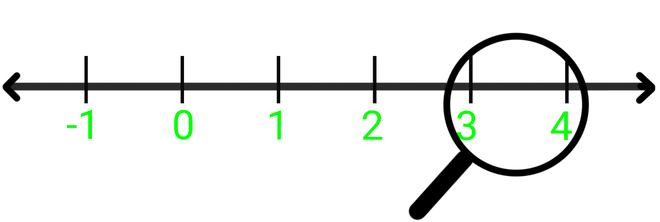Magnify 3 and 4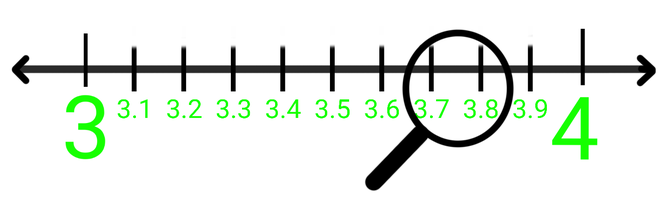Step 2: Now 3.76 will lie between 3.7 and 3.8, so we will magnify the value between 3.7 and 3.8.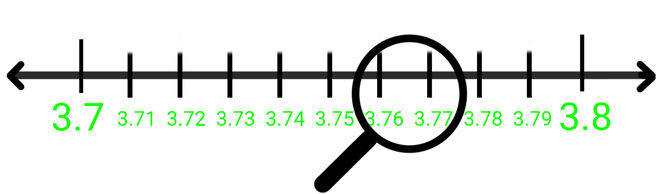Step 3: Now as we will magnify we will check the range, that is, 3.765 will lie between 3.76 and 3.77.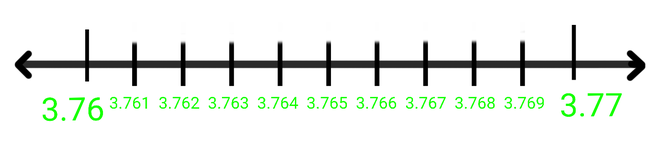Step 4: Now as we will magnify the value between 3.76 and 3.77 we will find the value 3.765.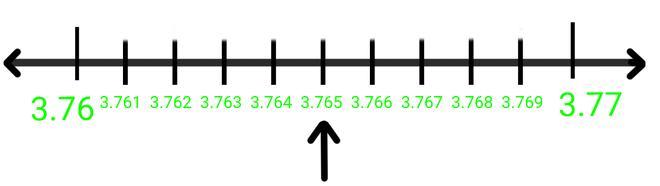Hence 3.765 is found between 3.76 and 3.77

### Question 2. Visualizeon the number line, up to 4 decimal places.

Now in this question, we have to represent the numberon the number line. The bar sign (   ͞   ) above .26 depicts that the number .26 is repeated continuously after the decimal point.  But it is given that we have to represent the number up to 4 decimal places only, that is, we have to represent 4.2626 on the number line.

Step 1: We will check the range of the number and magnify it accordingly.

Step 2: Now 4.2626 will lie between 4 and 5, so we will magnify the value between 4 and 5.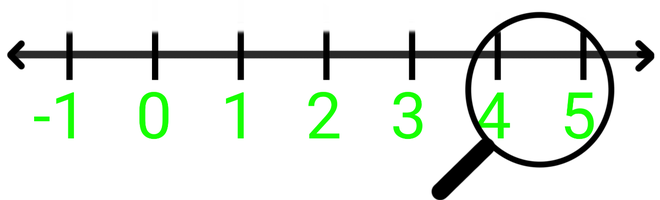Magnify 4 and 5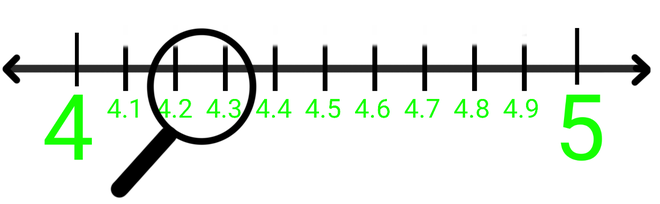Step 3: 4.26 will lie between 4.2 and 4.3, so we will magnify the value between 4.2 and 4.3.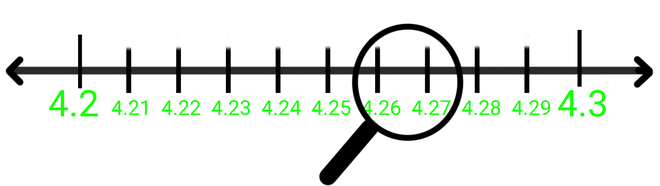Step 4: 4.262 will lie between 4.26 and 4.27, so we will magnify the value between 4.26 and 4.27.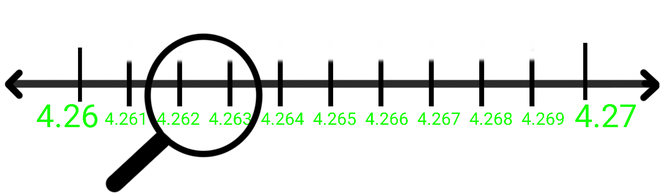Step 5: Now at last 4.2626 will lie between 4.262 and 4.263, so we will magnify the value between 4.262 and 4.263.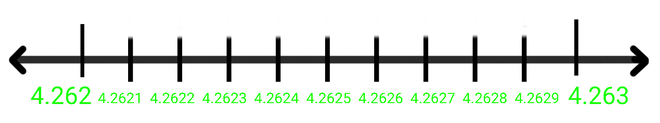Step 6: As we will magnify the value between 4.262 and 4.263 we will get 4.2626.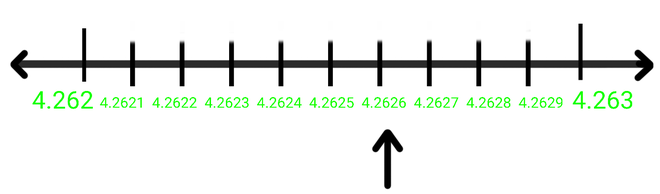Hence 4.2626 is found between 4.262 and 4.263

My Personal Notes arrow_drop_up
Related Articles